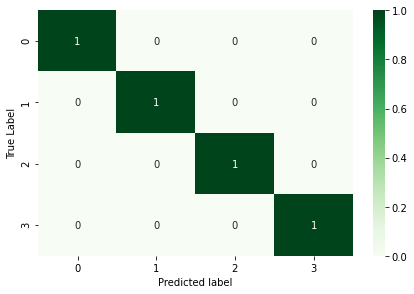# How did the Deep Learning model achieve 100% accuracy?

Original article was published on Deep Learning on Medium# How did the Deep Learning model achieve 100% accuracy?

It is important to look through the datasets before solving a Deep Learning problem. This article will take you through a scenario where the test set and validation set contains the same data as that of the training set. Have you ever wondered if this can happen? Well there are possibilities that datasets can be biased and this article is a result of my personal experience with one such problem.

## Introduction

The data set used here is a subset of food-101 data set, and can be found in TensorFlow datasets. My aim is to classify the images into four categories ‘chicken_curry, hamburger, omelette and waffles’. The data set consists of 8,345 images belonging to four classes and is divided in the following way:
Training set: 4000 images belonging to 4 classes
Validation set: 2217 images belonging to 4 classes
Testing set: 2128 images belonging to 4 classes

With this knowledge of the problem, let’s get started.

## ResNet50 Pre-Trained CNN

It is a deep residual network and the number ‘50’ refers to the depth of the network, meaning the network is 50 layers deep. It belongs to a sub-class of Convolution Neural Network. The network has over 23 million trainable parameters. ResNet-50 came into existence to solve the problem of vanishing gradients. ResNet-50 uses a skip connection where the input is added to the output of the original block and this mitigates the problem of vanishing gradient. You can also refer to the original research paper for more details. I assume that you are aware of the architecture if you have chosen to implement a deep learning solution using ResNet. The ResNet-50 model consists of 5 stages with each stage having a convolution and an identity block, where each Convolution block has three convolution layers and each identity block also has three convolution layers. The ResNet-50 model looks this:

## Transfer Learning

It is a method of training the data where the model has already been trained earlier on a large data set and has been used for classifying huge number of classes. ResNet-50 has been trained on ImageNet database consisting of millions of images and the weights are saved. These weights can be used while implementing the Transfer learning approach. There are two ways of using Transfer Learning, Feature-Extraction and Fine-Tuning. The solution to the design problem here focuses on the fine-tuning approach. In the fine-tuning approach, a fully connected layer is added on the top of the pre-trained ResNet-50 base model. The entire pre-trained model is frozen, which means the weights are not updated, and the learning process is halted. The fully connected layer added on the top of ResNet-50 base is trained for small number of epochs and then the 5c block of ResNet-50 pre-trained model is set trainable by unfreezing the relevant layers. The layers which are unfroze, in conjunction with the fully connected layer added are trained and used for training the data to obtain the classification result. The initial layers of a Convolution Neural Network learn the low-level features and as the network goes deep, higher level features are learnt. Hence, the higher layers are unfroze and further used in the training process. The dimension requirement for ResNet-50 model is (224,224,3). Hence, all the images are converted or resized to this dimension before feeding into the pre-trained model.

Having understood the concept of fine-tuning based transfer learning approach to image classification, I am moving ahead to the core of this article.